# Project 1 - Matlab

The project assignment should be solved individually. Each student should have his/her own solution and be prepared to explain and motivate any part of it. Please refer to the code of honor for more information.

# Description

This project will teach you about using transformation matrices, the perspective camera model and control of a robot arm. The setting is a 3 degree of freedom (DOF) robot arm.

# Part I: Coordinate transforms

In the first part you will derive the transformation matrices for the different parts of the arm so that you can easily move between different coordinate systems. The figure below shows the arm with coordinate systems attached to the three joints (red) and the end effector (blue).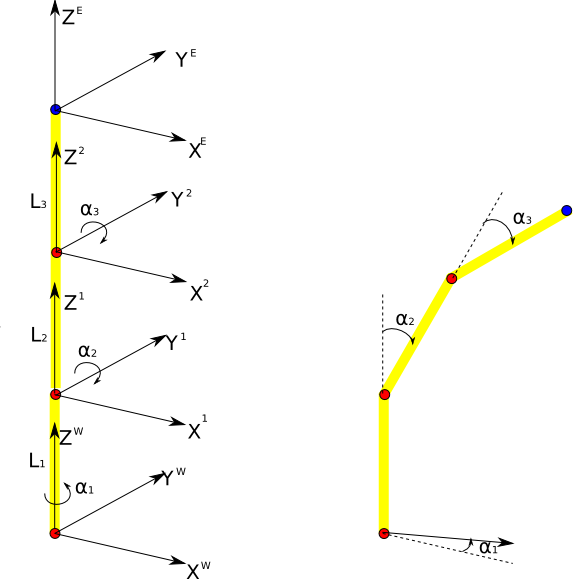Corresponding 3D visualization of the joints of our 3 DOF robot arm.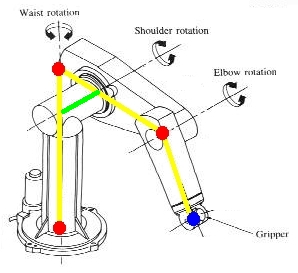Note that the robot depicted here is in fact a little more complicated, by having an offset translation (light green) between two joints. In the problem that should be handled here we abstract from this offset, and assume that all joints are lying on one plane in 3D space. Additional info: joint 1 is rotating around its own Z-axis (`cylindrical' joint), while joints 2 and 3 are rotating around their Y-axes (`rotational' joint).

When using the arm for picking up things and otherwise interacting with the world it is often convenient to express the position of objects in the end effector frame. Other times it is easier to do so using the world coordinate system. In general you might want to be able to express a certain position in any given frame.

Let us consider a vector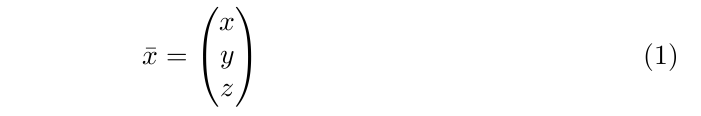If two coordinate systems 1 and 2 are related by a pure rotation around some axis then you can transform a coordinate expressed in the second (rotated) coordinate system into the first one using the following relation which involves a rotation matrix (see Wikipedia page about rotation matrix). The rotation matrix for rotations around x,y,z axes are shown below.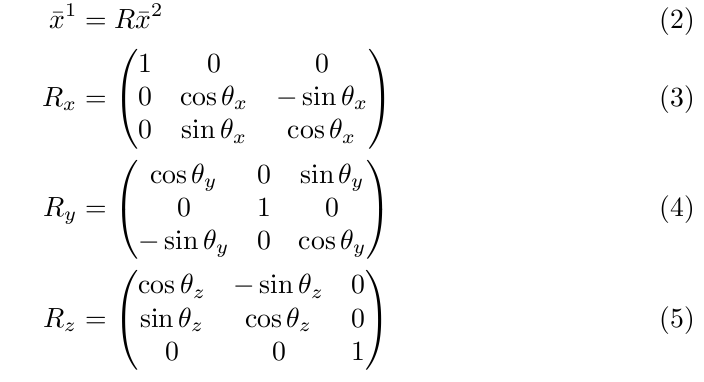If instead the two coordinate systems are related by a pure translation then the relationship will be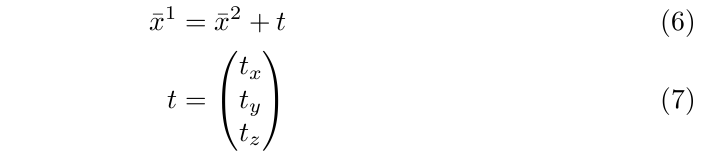We can combine the rotation with a translation (expressed in frame 1) and then get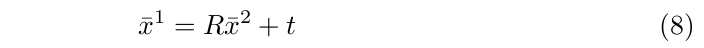By introducing so called homogenous coordinates (pad the original vector with a 1)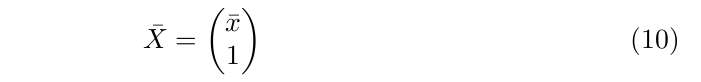we can write the above expression using a single matrix, the so called transformation matrix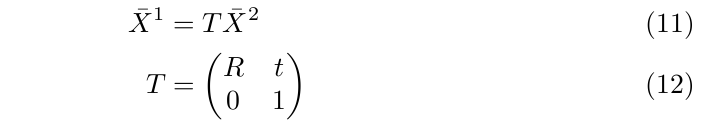An example of a transformation matrix for the case of a rotation around the z-axis and a translation is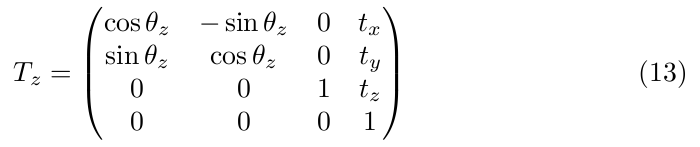One thing that makes these transformation matrices so handy is one can multiply them in a chain to combine several transformations. If we consider two different transformations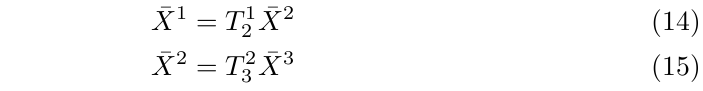we can combine them to get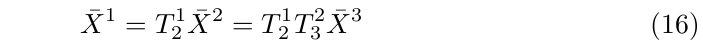from which we easily can identify the transformation from the 1st frame to the 3rd.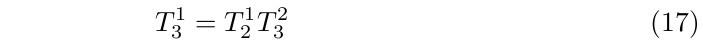If we want to get the transformation the other way around we can simply invert the expression and get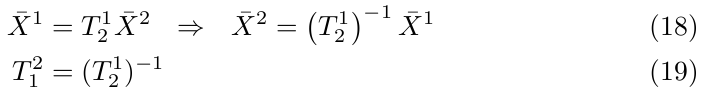# Part II: Arm control

In the second part we will look at how to control the arm so that the end effector reaches some desired position. The simplest form of controller is the so called proportional controller (or P-controller). In this case we want to control the world position of the end effector to some reference position.

The general structure for a proportional controller is that we let the control signal be proportional to the error. We thus calculate the error, e, between the reference position and the current position and multiply this with some constant Kp which is called the controller gain.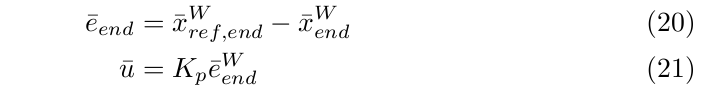Notice that in our case the error is a vector consisting of the x,y,z values. We could imagine using controller gain matrix but here we will be using a scalar gain, i.e. the same gain for all directions in space.

In our setup we want to control the speed of the end effector in world coordinates. This means that the control signal (u) is this end effector speed which thus will be proportional to the position error. This in turn means that the larger the error the higher the speed and vice-versa which makes intuitive sense. Below we will use the Newton's notation to denote time derivatives (e.g. speed):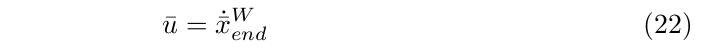However, we cannot directly control the position of the end effector, all we can control is the joint angles. We stack those together and form the joint angle vector.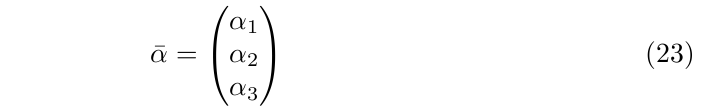Using the transformations discussed in Part I we can express the position of the end effector in the world coordinate system using a transformation from the end effector frame to the world frame and remembering that the end effector frame is located at the end effector which corresponds to position (0,0,0) in that frame. Using this we can get the so called forward kinematics (see Wikipedia page about forward kinematics) which expresses the end effector position in terms of the joint angles. In the equations below the notation 1:3 refers to picking the first 3 rows of the vector of values of function F (we omit the 4th value equal to 1).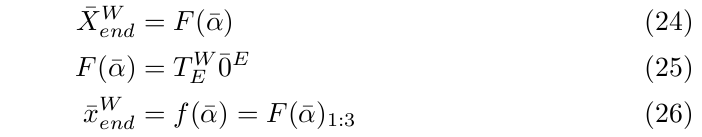We can now relate the speed in Cartesian coordinate with the speed in joint space which is where we can actually apply control. We do this by derivating the forward kinematics to get the Jacobian, J.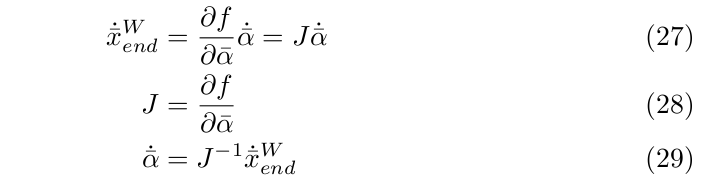Finally, we can put all the pieces together to get the P-controller that uses the error in the end effector position expressed in Cartesian world coordinates and calculates joint angle speeds to apply to the arm.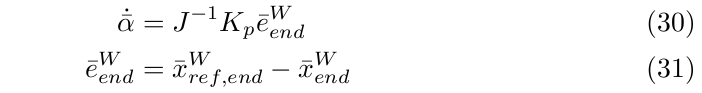# Part III: Camera projection

In the last part we will look at the perspective camera model and how points in the world are projected into the image. The figure below illustrates how the image plane coordinates are related to the position of a point in the world. There are three coordinate systems involved here. First we have the camera coordinate system (C) where the z-axis typically points along the optical axis. Secondly, we have two different image coordinates. Notice that the image defines a mapping from 3D to 2D.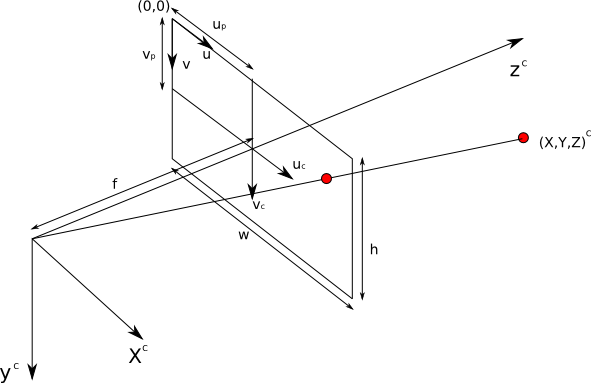The first coordinate system is centred around the optical axis (uc,vc). The relation with the world coordinates involves the focal length f. The position of the optical axis in the image is given by the principle point (up,vp) expressed in the image coordinate system (u,v) which is centred in the upper left corner of the image.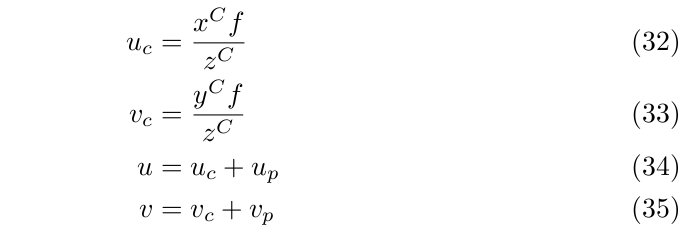In an ideal camera the principle point would be at the centre of the image, i.e. be given by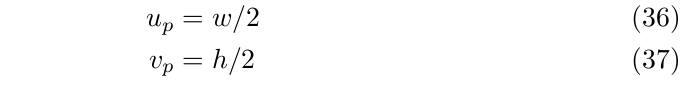where w and h are the width and height of the image respectively.

The height and width of the image and the focal length are expressed in pixels. More elaborate models would also need to take into account various forms of distortion, pixels not having the same dimensions in both directions, etc.

Obviously if the object that you try to see with the camera is behind the image plane it cannot be detected. Also it can only be detected if it is projected with in the image boundaries, i.e., 0 < u < w and 0 < v < h

# Requirements

Part 1
• Derive the transformation between the three other coordinate systems (1,2 and E) and the world.
• Write a function that returns the position of the joints and end effector based on the current joint angles. This is the so called forward kinematics of the arm.
• Make a function that allows you to visualise the arm given the output of the forward kinematics (previous question) which in turn depends on the joint angles (alpha 1,2,3). Label the axis so that one can tell x,y,z apart easily. The function should allow you to feed in an optional argument (vector of joint angles) so that it can show the current joint angles in the title of the figure (in degrees). Let L1=1.3, L2=1.2, L3=1.1
• Display the robot moving in 3D using the the following file for the joint angles. The file contains three columns, corresponding the alpha1,2,3 respectively.
• Display the trajectories of the joints positions and the end effector in 3D (that is showing how it moved from start to to end of motion).
• Display the position of the end effector throughout the motion projected down on the xy-plane.

Part 2
• Write a function so that you can simulate motion of the arm. You can ignore inertia, dynamics, couplings, etc and assume that each joint are independent and that the angles are governed by:
alpha(i)_{t+1} = alpha(i)_t + dt * speed(i)
where alpha(i)_t is the angle of joint i at time t and speed(i) is the angle speed. Start with dt=0.1.
• Write a script that uses your arm simulator to move the arm around to make sure that it works. Try for example
alpha = [90,30,30]’*pi/180
and
speed = [5,3,1]*pi/180
as starting values and run the simulation as long as alpha(i) is between pi and -pi for any i.
• Design a controller that allows you to drive the robot to a given end effector position. Start with initial configuration
alpha = [0,30,30]’*pi/180
and let the goal position for the end effector be [1.5,1.5,1].
• Display the joint angles and speed as a function of time. Display the end effector position as a function of time. Make sure its easy to interpret the plots.

Part 3

Assume that we have mounted the camera dead-centre on the end effector and that the camera coordinates are defined as in the figure below, i.e., its rotated around the z-axis of the end effector reference frame.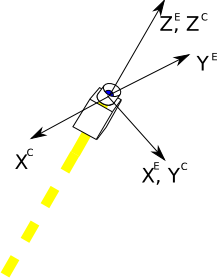• Create a function that returns the image space position (u,v) for a point in world coordinates (x,y,z) given the focal length of the camera, joint angle vector and transformation between end effector and camera frame.
• Assume that the camera parameters are, width = 640, height = 480 and focal length = 300. Further, assume the arm is in configuration [30,30,30] (degrees). How does a point move in image space (u,v) if it moves according to this file. The file contains one column for x,y and z. Assume that the optical axis goes through the center of the image (i.e. that the principle point is (width/2,height/2).

Together with the code you should submit a document that clearly states how to execute the different parts and explains the derivations of the different transforms.

Good Luck!